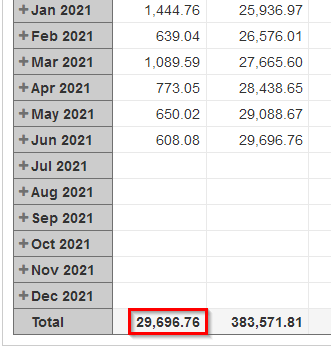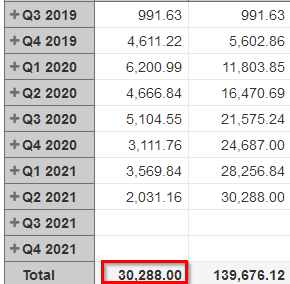# eazyBI total not correct

Hello,
in some of my reports I have the problem, that the built in total on columns is not correct when time dimension is set to show on monthly basis. If I change the dimension to quarterly for example, it shows a correct sum.
Here an example for the wrong sum:and here for the correct sum:The sum is on a calculated member I created. Any help is much appreciated.

The “Total” function is optimized for improved performance. In some cases, with calculated members and measures, this produces wrong results. To overcome this, please update the formula of the measure to look similar to the one below:

``````CASE WHEN [Time].CurrentHierarchyMember.Level.Name <> '\$total_aggregate'
THEN
...
END
``````

Replace the `...` part with the formula you are using right now. This will override the “Total” function optimization part and force it to sum all the values on rows.

Best,
Roberts // eazyBI support

@roberts.cacus I tried with this method but it is not working.

Share the formula you used.

Best,